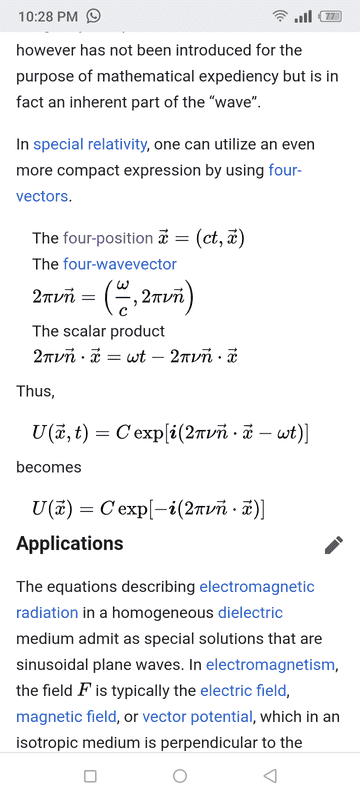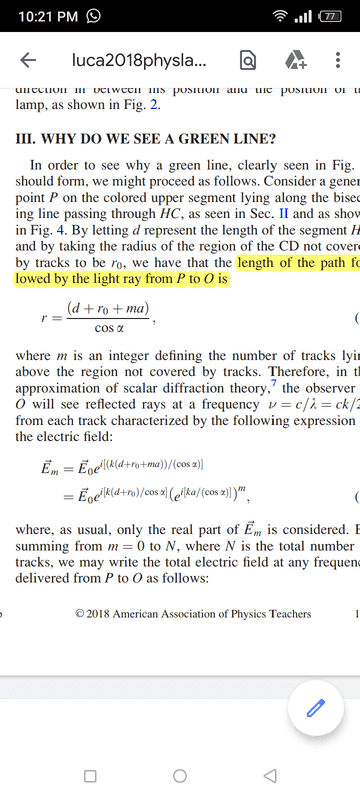# Electromagnetic wave equation - phase and amplitude

how we get "e^(ikr)"...?
Plz ans me... Thanks

how we get "e^(ikr)"...?
Plz ans me... Thanks
Though it's e^(e(x-ct))

Nugatory
Mentor
how we get "e^(ikr)"...?
Though it's e^(e(x-ct))
There's no ##r## in the discussion above, so it's not clear what you're asking about when you write ##e^{(ikr)}##, nor does ##e^{e(x-ct)}## make sense in the context of this discussion.

Are you familiar with the general solution of the wave equation? It's a differential equation so we solve it by making an educated guess as to the general form of the solution (in this case, ##Ae^{i(\omega t-kx)}##), plugging it into our differential equation, and then solving for for the constants ##k## and ##\omega##. Do it right, and you'll get an equation describing a wave travelling at speed ##c##; post #6 by @RPinPA tells you how to interpret it.

(Once you've clarified your question, it may make sense to move it to a thread of its own)

sir plz look at the electric field expression...
They are writing E°e^(ikr). The author puts r value...
I have searched a lot for it and I found its solution....
But i found that they calculate it with a very different method... They calculate 2pifn. x= wt-2pifn.x...
You can see in the image below...
So plz guide mehere is the screen shot sir...!!

caz
Gold Member
sir plz look at the electric field expression...
They are writing E°e^(ikr). The author puts r value...
I have searched a lot for it and I found its solution....
But i found that they calculate it with a very different method... They calculate 2pifn. x= wt-2pifn.x...
You can see in the image below...
So plz guide meView attachment 280809
Instead of treating space as a vector and time as a scalar, they are using the relativistic approach of a four-vector which combines the two into a single vector with 4 terms. Notice that the inner product is positive for the time term and negative for the space term. Some use the opposite sign convention for the inner product so one should be careful.

caz
Gold Member